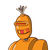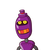# The length of a rectangle in twice its breadth . If the perimeter is 36 meters , find the length and breadth of the rectangle. January 5, 2022 by Amaya

The length of a rectangle in twice its breadth . If the perimeter is 36 meters , find the length and breadth of the rectangle.

Anyone ?​

### 2 thoughts on “The length of a rectangle in twice its breadth . If the perimeter is 36 meters , find the length and breadth of the rectangle.<br”

1.Let length and breadth be 2x and x respectively

Perimeter of Rectangle = 2 × ( l + b )

=> 36 = 2 × ( 2x + x)

=> 36 = 2 × 3x

=> 36 = 6x

=> 36/6 = x

=> 6 = x

or x = 6m

2.Let length and breadth of the rectangle be 2x and x respectively

Perimeter of Rectangle = 2 × ( l + b )

=> 36 = 2 × ( 2x + x )

=> 36 = 2 × 3x

=> 36 = 6x

=> 36/6 = x

=> 6 = x

or x = 6m